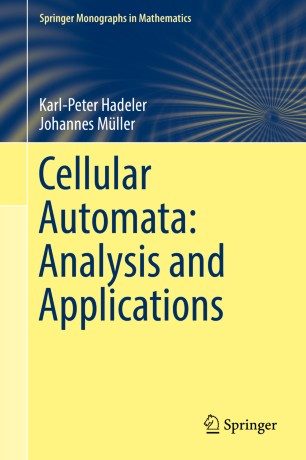# Cellular Automata: Analysis and Applications

• Johannes MüllerBook

Part of the Springer Monographs in Mathematics book series (SMM)

1. Front Matter
Pages i-xi
Pages 1-17
Pages 19-35
Pages 37-73
Pages 75-109
Pages 111-153
Pages 155-177
Pages 179-195
Pages 197-252
Pages 253-285
Pages 287-334
Pages 335-375
Pages 377-403
Pages 405-425
15. Back Matter
Pages 427-467

### Introduction

This book focuses on a coherent representation of the main approaches to analyze the dynamics of cellular automata. Cellular automata are an inevitable tool in mathematical modeling. In contrast to classical modeling approaches as partial differential equations, cellular automata are straightforward to simulate but hard to analyze. In this book we present a review of approaches and theories that allow the reader to understand the behavior of cellular automata beyond simulations.
The first part consists of an introduction of cellular automata on Cayley graphs, and their characterization via the fundamental Cutis-Hedlund-Lyndon theorems in the context of different topological concepts (Cantor, Besicovitch and Weyl topology). The second part focuses on classification results: What classification follows from topological concepts (Hurley classification),  Lyapunov stability (Gilman classification), and the theory of formal languages and grammars (Kůrka classification). These classifications suggest to cluster cellular automata, similar to the classification of partial differential equations in hyperbolic, parabolic and elliptic equations. This part of the book  culminates in the question, whether properties of cellular automata are decidable. Surjectivity, and injectivity are examined, and the seminal Garden of Eden theorems are discussed.
The third part focuses on the analysis of cellular automata that inherit distinct properties, often based on mathematical modeling of biological, physical or chemical systems. Linearity is a concept that allows to define self-similar limit sets. Models for particle motion show how to bridge the gap between cellular automata and partial differential equations (HPP model and ultradiscrete limit). Pattern formation is related to linear cellular automata, to the Bar-Yam model for Turing pattern, and Greenberg-Hastings automata for excitable media. Also models for sandpiles, the dynamics of infectious diseases and evolution of predator-prey systems are discussed. Mathematicians find an overview about theory and tools for the analysis of cellular automata. The book contains an appendix introducing basic mathematical techniques and notations, such that also physicists, chemists and biologists interested in cellular automata beyond pure simulations will  benefit.

### Keywords

cellular automata classification of cellular automata dynamics of cellular automata applications of cellular automata decision problems in cellular automata